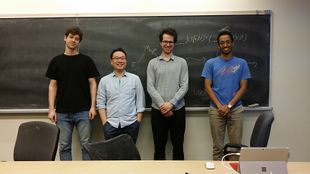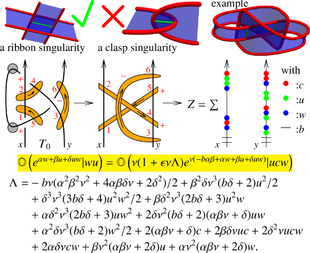Back to AKT-17.

# Week of... Notes and Links
: The Jones polynomial via the Kauffman bracket, following 170110-JonesPoly.nb (pre-class: pdf, nb; post-class: pdf, nb).
: Knots, algebras, Yang-Baxter, CYBE, Lie algebras, universal enveloping algebras, formulas.
2 Jan 16 : More on the Jones polynomial via the Kauffman bracket, following 170117-MoreJones.nb (pre-class: pdf, nb; post-class: pdf, nb).
: Continuation, 170117-FastSlowRace.nb (pre-class: pdf, nb; post-class: pdf, nb), knot genus.
: The Lie algebra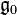${\mathfrak g}_0$ following 170120-g0.nb (pre-class: pdf, nb; post-class: pdf, nb).
3 Jan 23 : Tangles and meta-monoids.
: The fundamental group, meta-Hopf algebras, algebraic knot theory.
: The Lie algebra${\mathfrak g}_0$ following 170127-g0.nb (pre-class: pdf, nb; post-class: pdf, nb).
Class Photo.
4 Jan 30 : Tangles, links, and 3-manifolds.
: Genus, ribbon, slice.
: Normal orderings following 170203-g0dsO.nb (pre-class: pdf, nb; post-class: pdf, nb).
5 Feb 6 :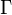$\Gamma$-calculus following 170207-GammaCalculus.nb (pre-class: pdf, nb; post-class: pdf, nb), part I.
:$\Gamma$-calculus following 170207-GammaCalculus.nb (pre-class: pdf, nb; post-class: pdf, nb), part II.
: The main${\mathfrak g}_0$ theorem following 170210-g0MainTheorem.nb (pre-class: pdf, nb; post-class: pdf, nb).
6 Feb 13 : Expansions in general; also using 170214-ProgressiveScanning.nb (pre-class: pdf, nb; post-class: pdf, nb).
: Expansions for tangles, finite type invariants.
: Computing the${\mathfrak g}_0$ invariant following 170217-g0Invariant.nb (pre-class: pdf, nb; post-class: pdf, nb).
7 Feb 27 : Expansions and the fundamental theorem for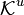${\mathcal K}^u$.
: Algebraic structures on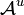${\mathcal A}^u$.
: Conclusion of the${\mathfrak g}_0$ discussion following 170303-g0LemmaAndInvariant.nb (pre-class: pdf, nb; post-class: pdf, nb).
8 Mar 6 : The polished${\mathfrak g}_0$ invariant following 170307-g0Polished.nb (pre-class: pdf, nb; post-class: pdf, nb) and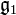${\mathfrak g}_1$ lemmas following 170307-geps.nb (pre-class: pdf, nb; post-class: pdf, nb).
: The logos for${\mathfrak g}_1$ following 170307-geps.nb (pre-class: pdf, nb; post-class: pdf, nb).
: Algebraic structures on${\mathcal A}^u$, trivalent diagrams.
9 Mar 13 Monday is the last day to drop this class.
: Deriving and testing the Logos following 170317-g1Invariant.nb (pre-class: pdf, nb; post-class: pdf, nb) and 170317-TestingTheLogos.nb (pre-class: pdf, nb; post-class: pdf, nb).
10 Mar 20 : The${\mathfrak g}_1$ invariant following 170321-g1Invariant.nb (pre-class: pdf, nb; post-class: pdf, nb) and 170321-Polishedg1Invariant.nb (pre-class: pdf, nb; post-class: pdf, nb).
Tuesday Hour 2: offline, though see BBS/AKT17-170321-145350.jpg and BBS/AKT17-170321-150540.jpg.
: Associators, also following 170324-Associator.nb (pre-class: pdf, nb; post-class: pdf, nb) and 170324-MutipleZetaValues.pdf.
11 Mar 27 Classes canceled due to an MSRI workshop.
12 Apr 3 : Diagrams to Universal Enveloping Algebras, the u-case.
: Virtual knots and diagrams to Universal Enveloping Algebras, the v-case.
: Sjabbo Schaveling: The Quantum Double towards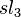$sl_3$, following 170407-Classical_Algebra_E0.nb (pdf, nb), 170407--Quantum_Algebra_E0.nb (pdf, nb), and 170407--E_machten_Algebra_E0_v2.nb (pdf, nb).